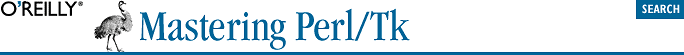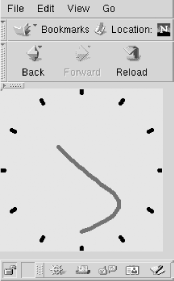home | O'Reilly's CD bookshelfs | FreeBSD | Linux | Cisco | Cisco Exam## C.11. clock-bezier.ppl

In Chapter 22, "Perl/Tk and the Web", we discussed the Netscape PerlPlus Plugin and used several PPL programs in our examples. We include two PPL programs in this appendix. The first is the clock-bezier.ppl program, shown in Figure C-3.#### Figure C-3. clock-bezier.ppl

```#!/usr/local/bin/perl -w
#
# This most entertaining program was written in Tcl/Tk by Scott Hess
# (shess@winternet.com).  It's a clock that uses a bezier curve anchored
# at four points—the hour position, the minute position, the second
# position and the center of the clock—to show the time.
#
# <Button-1> switches between display modes, and <Button-2> switches
# between line thicknesses.
#
# Perl/Tk version by Stephen.O.Lidie@Lehigh.EDU, 2000/02/05.

use POSIX qw/asin/;
use Tk;
use subs qw/buildclock hands setclock/;
use vars qw/\$clock %hand \$mw \$pi180 \$resize/;
use strict;

%hand = (
hour    => 0.40,
minute  => 0.75,
second  => 0.85,
0       => 0.00,
intick  => 0.95,
outtick => 1.00,
width   => 0.05,
scale   => 100,

type    => 'bezier',
types   => [qw/normal curve angle bezier/],
tindx   => 3,
normal  => [qw/minute 0 0 second 0 0 hour 0 0 minute/],
curve   => [qw/minute 0 second 0 hour 0 minute/],
angle   => [qw/minute second second hour/],
bezier  => [qw/minute second 0 hour/],

tick    => [qw/intick outtick/],
);
\$pi180 = asin(1) / 90.0;
\$resize = 0;

\$mw = MainWindow->new;
\$clock = \$mw->Canvas(qw/-width 200 -height 200/);
\$clock->pack(qw/-expand 1 -fill both/);
\$mw->bind('<Configure>' => \&buildclock);
\$mw->bind('<Button-1>'  => \&incrtype);
\$mw->bind('<Button-2>'  => \&incrwidth);
buildclock;
\$mw->repeat(1000 => sub {my(@t) = localtime; setclock @t[0 .. 2]});
MainLoop;

sub buildclock  {

# Build the clock.  Puts tickmarks every 30 degrees, tagged
# "ticks", and prefills the "hands" line.

my \$pi180 = asin(1)/90.0;

Tk::catch {\$clock->delete('marks')};
\$clock->update;
my \$w = \$clock->width;
\$mw->geometry("\${w}x\${w}") if \$resize; # ensure clock is square
\$resize++;
\$hand{scale} = \$w / 2.0;

# This is a horrid hack.  Use the hands( ) procedure to
# calculate the tickmark positions by temporarily changing
# the clock type.

my \$type = \$hand{type};
\$hand{type} = 'tick';
my %angles;
for (my \$ii = 0; \$ii < 12; \$ii++) {
\$angles{intick} = \$angles{outtick} = \$ii * 30 * \$pi180;
\$clock->createLine(hands(\%angles), -tags => [qw/ticks marks/]);
}
\$hand{type} =  \$type;

\$clock->createLine(qw/0 0 0 0 -smooth 1 -tags/ => [qw/hands marks/]);
\$clock->itemconfigure(qw/marks -capstyle round -width/ =>
\$hand{width} * \$hand{scale});
}

sub hands {

# Calculate the set of points for the current hand type and
# the angles in the passed array.

my(\$aa) = @_;

my \$ss = \$hand{scale};
my @points;
foreach my \$desc ( @{ \$hand{\$hand{type}} } ) {
push @points, sin(\$aa->{\$desc}) * \$hand{\$desc} * \$ss + \$ss;
push @points, \$ss - cos(\$aa->{\$desc}) * \$hand{\$desc} * \$ss;
}
#print join(', ', @points), "\n";
return @points;
}

sub incrtype {
\$hand{type} = \$hand{types}->[ ++\$hand{tindx} % @{\$hand{types}} ];
}

sub incrwidth {
my \$w = \$hand{width} + .05;
\$hand{width} = \$w > .25 ? 0 : \$w;
\$clock->itemconfigure('marks', -width => \$hand{width} * \$hand{scale});
}

sub setclock {

# Calculate the angles for the second, minute, and hour hands,
# and then update the clock hands to match.

my(\$second, \$minute, \$hour) = @_;

my %angles;
\$angles{0}      = 0;
\$angles{second} = \$second *  6 * \$pi180;
\$angles{minute} = \$minute *  6 * \$pi180;
\$angles{hour}   = \$hour   * 30 * \$pi180 + \$angles{minute} / 12;

my \$sector  = int( \$angles{second} + 0.5 );
my(@colors) = qw/cyan green blue purple red yellow orange/;
\$clock->itemconfigure(qw/hands -fill/ => \$colors[\$sector]);

\$clock->coords('hands',  hands \%angles);
}```